AP State Syllabus AP Board 8th Class Maths Solutions Chapter 5 Comparing Quantities Using Proportion Ex 5.2 Textbook Questions and Answers.

## AP State Syllabus 8th Class Maths Solutions 5th Lesson Comparing Quantities Using Proportion Exercise 5.2Question 1.
In the year 2012, it was estimated that there were 36.4 crore Internet users worldwide. In
the next ten years, that number will be increased by 125%. Estimate the number of Internet
users worldwide in 2022.
Solution:
Internet users in the year 2012
= 36.4 crores.
The number will be increased by next
10 years = 125%
∴ The no. of internet users in the year 2022
= 36.4 + 125% of 36.4
= 36.4 + $$\frac { 125 }{ 100 }$$ × 36.4
= 36.4 + 45.5
= 81.9 crores.Question 2.
A owner increases the rent of his house by 5% at the end of each year. If currently its rent is ₹ 2500 per month, how much will be the rent after 2 years’?
Solution:
Present house rent = ₹ 2500 If the owner increases the rent by 5% on every year then the rent of the house after 2 years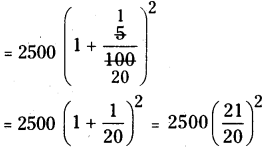= 2500 × $$\frac { 21 }{ 20 }$$ x $$\frac { 21 }{ 20 }$$
= ₹ 2756.25

Question 3.
On Monday, the value of a company’s shares was ₹ 7.50. The price increased by 6% on Tuesday, decreased by 1.5% on Wednesday, and decreased by 2% on Thursday. Find the value of each share when trade opened on Friday.
Solution:
The value of the share when trade opened on Friday
= ₹ 7.674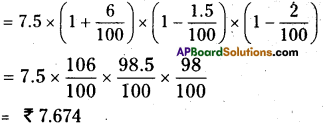Question 4.
With most of the Xerox machines. you can reduce or enlarge your original by entering a percentage for the copy. Reshma wanted to enlarge a 2 cm by 4 cm drawing. She set the Xerox machine for 150% and copied her drawing. What will be the dimensions of the
copy of the drawing be’?
Solution:
Length of the copy = 2 cm
If the length is increase in 150% then its
measure = 150 % of 2 cm
= $$\frac { 150 }{ 100 }$$ × 2 = 1.5 × 2 = 3 cm

If the breadth is increase in 150% then
its measure = 150 % of 4 cm 150
= $$\frac { 150 }{ 100 }$$ × 4 = 1.5 × 4 = 6 cm
100
∴ New length = 3 cmQuestion 5.
The printed price of a book is ₹ 150. And discount is 15%. Find the actual amount to be paid.
Solution:
The printed price of a book = ₹ 150
Discount % = 15%
∴ Discount = 15% of 150
= $$\frac { 15 }{ 100 }$$ × 150 = ₹22.5
∴ The C.P. of a book = 150 – 22.5
= ₹127.50/-

Question 6.
The marked price of an gift item is ₹ 176 and sold it for ₹ 165. Find the discount percent.
Solution:
Marked price of a gift = ₹176
S.P. = 165
Discount = 176 – 165 = ₹ 11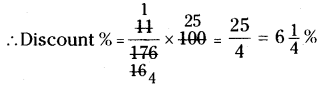Question 7.
A shop keeper purchased 200 bulbs for ₹10 each. However 5 bulbs were fused and put
them into scrap. The remaining were sold at ₹12 each. Find the gain or loss percent.
Solution:
The C.P. of 200 bulbs at the rate of ₹10 for each = 200 × 10 = ₹ 2000
If 5 bulbs are fused then remaining are
= 200 – 5 = 195
∴ TheS.P. of 195 bulbs at the rate of ₹12 for each = 195 × 12 = ₹ 2340
∴ S.P. > C.P.
∴ Profit = S.P.-C.P.
= 2340 – 2000 = 340
Profit = $$\frac { Profit }{ C.P }$$ × 100 = $$\frac { 340 }{ 2000 }$$ × 100
Profit = 17%

Question 8.
Complete the following table with appropriate entries (Wherever possible)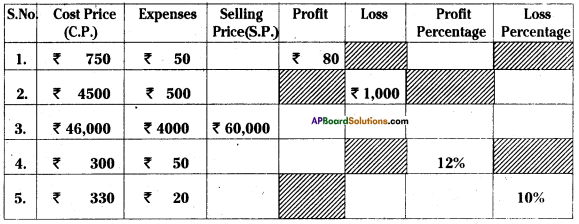Solution: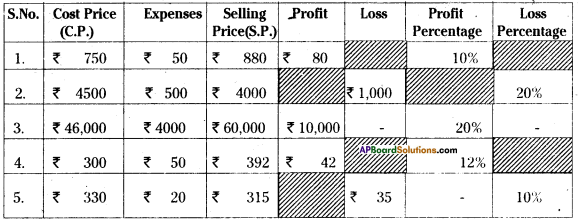Question 9.
A table was sold for ₹2,142 at a gain of 5%. At what price should it be sold to gain 10%.
Solution:
S.P. of a table = ₹ 2142
Profit = 5%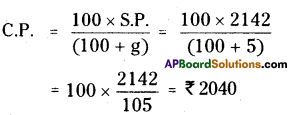∴ The C.P. of buyyer = ₹ 2040
Profit % = 10%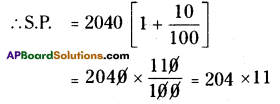∴ S.P. = ₹2244

Question 10.
Gopi sold a watch to Ibrahim at 12% gain and Ibrahim sold it to John at a loss of 5%. If John paid ₹1,330, then find how much did Gopi sold it?
Solution:
. Let Gopi’s cost price = ₹100
Gain = 12%
∴ Gopi’s selling price to Ibrahim or Ibrahim’s cost price = ₹100 + ₹12 = ₹112
∴ Ibrahim’s loss = 5%
∴ Ibrahim’s selling price =
$$112\left(\frac{100-5}{100}\right)=\frac{112 \times 95}{100}$$ = ₹106.40
For ₹100 we get = ₹106.40
For ₹1330 how much we get ?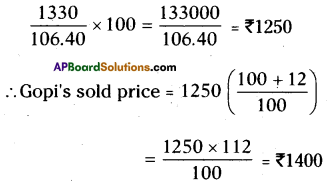Question 11.
Madhu and Kavitha purchased a new house for ₹3,20,000. Due to some economic
problems they sold the house for ₹2,80,000. Find (a) The loss incurred (b) the loss percentage.
Solution:
C.P. of a house = ₹ 3,20,000
S.P. of a house = ₹ 2,80,000
∴ C.P. > S.P.
a) Loss
= C.P. – S.P.
= 3,20,000 – 2,80,000 = 40,000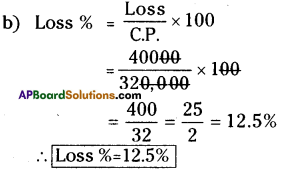Question 12.
A pre-owned car show-room owner bought a second hand car for ₹ 1,50,000. He spent ₹20,000 on repairs and painting, then sold it for ₹ 2,00,000. Find whether he gets profit or loss. If so, what percent?
Solution:
After repair, the C.P of a car
= 1,50,000 + 20,000 = 1,70,000
S.P. of a ear = ₹ 2,00,000
∴ S.P. > C.P.
∴ Profit = S.P.-C.P.
= 2,00,000-1,70,000= 30,000
Profit = $$\frac { Profit }{ C.P }$$ × 100
$$\frac { 30,000 }{ 1,70,000 }$$ × 100 = Profit = $$\frac { 300 }{ 17 }$$
Profit% = 17.64%Question 13.
Lalitha took a parcel from a hotel to celebrate her birthday with her friends. It was billed with ₹ 1,450 including 5% VAT. Lalitha asked for some discount, the hotel owner gave 8% discount on the bill amount. Now find the actual amount that lalitha has to pay to the hotel owner
Solution:
After allowing 5% VAT, the total bill = ₹ 1450
If 8% discount is allowed on bill, then
Discount = 8% of 1450
$$\frac { 8 }{ 100}$$ × 1450 = ₹116
Discount = ₹116
∴ Lalitha has to pay the bill = 1450 -116
= ₹ 1334

Question 14.
If VAT is included in the price, find the original price of each of the following.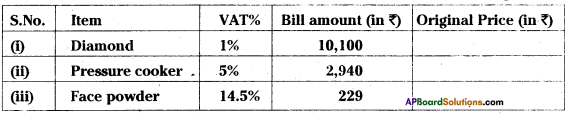Solution: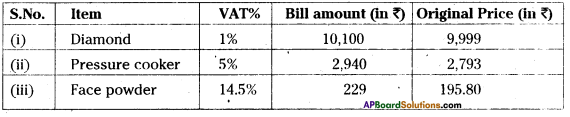Question 15.
Find the buying price of each of the following items when a sales tax of 5% is added on them.
(1) a towel of ₹50 (ii) Two bars of soap at ₹35 each.
Solution:
Given that Sales tax = 5%
(i) Cost of a towel = ₹ 50
Sales Tax = 5% of 50
= $$\frac { 5 }{ 100 }$$ x 50 = $$\frac { 5 }{ 2 }$$ = ₹ 2.50
∴ C.P. = Net Price + Sales
Tax = 50 + 2.50 = ₹ 52.50

(ii) The cost of two soaps at the rate of
₹ 35 each = 2 × 35 = ₹ 70
Sales Tax = 5% of 70
= $$\frac { 5 }{ 100 }$$ × 70 = $$\frac { 7 }{ 2 }$$ = ₹ 3.50
∴ C.P. = Net Price + Sales
Tax = 70 + 3.50 = ₹ 73.50Question 16.
A Super-Bazar prices an item in rupees and paise so that when 4% sales tax is added, no rounding is necessary because the result is exactly in ‘n’ rupees, where ‘n’ is a positive integer. Find the smallest value of ’n’.
Solution:
Let the cost price = x say
∴ If x is increased 4% sales tax is added then
x + 4% of x = n
x + $$\frac { 4 }{ 100 }$$ × x = n
$$\frac { 140x }{ 100 }$$ = n
x = x = n × $$\frac { 100 }{ 104 }$$ = $$\frac{25 \times \mathrm{n}}{26}$$
∴ n should be a least multiple of 26, then only the value of the article should be represented in only rupees.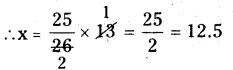[∵n = 13, 26, 39. from them 13 should betaken]
:. Required value of the article
=12.50 + $$\frac { 4 }{ 100 }$$ × 12.5
12.50 + 0.5 = ₹13